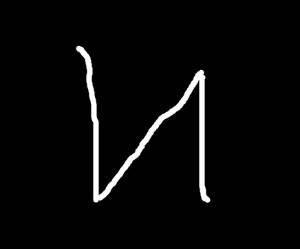# SONAR Based Obstacle Avoidance Robot

I implement a SONAR like system to provide autonomy to an old RC robot.

IntermediateShowcase (no instructions)369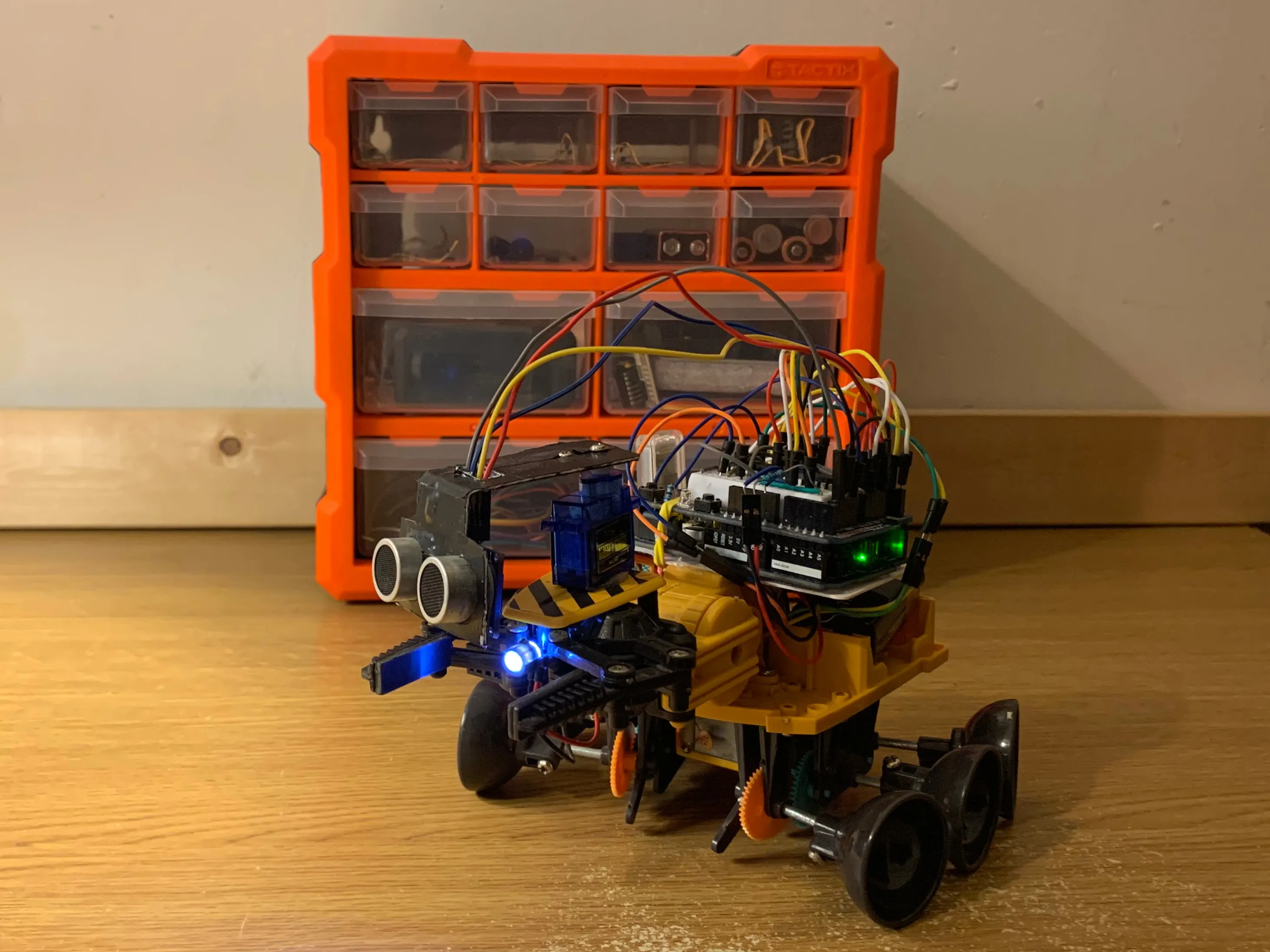## Things used in this project

### Hardware componentsArduino UNO & Genuino UNO
×1Jumper wires (generic)
×1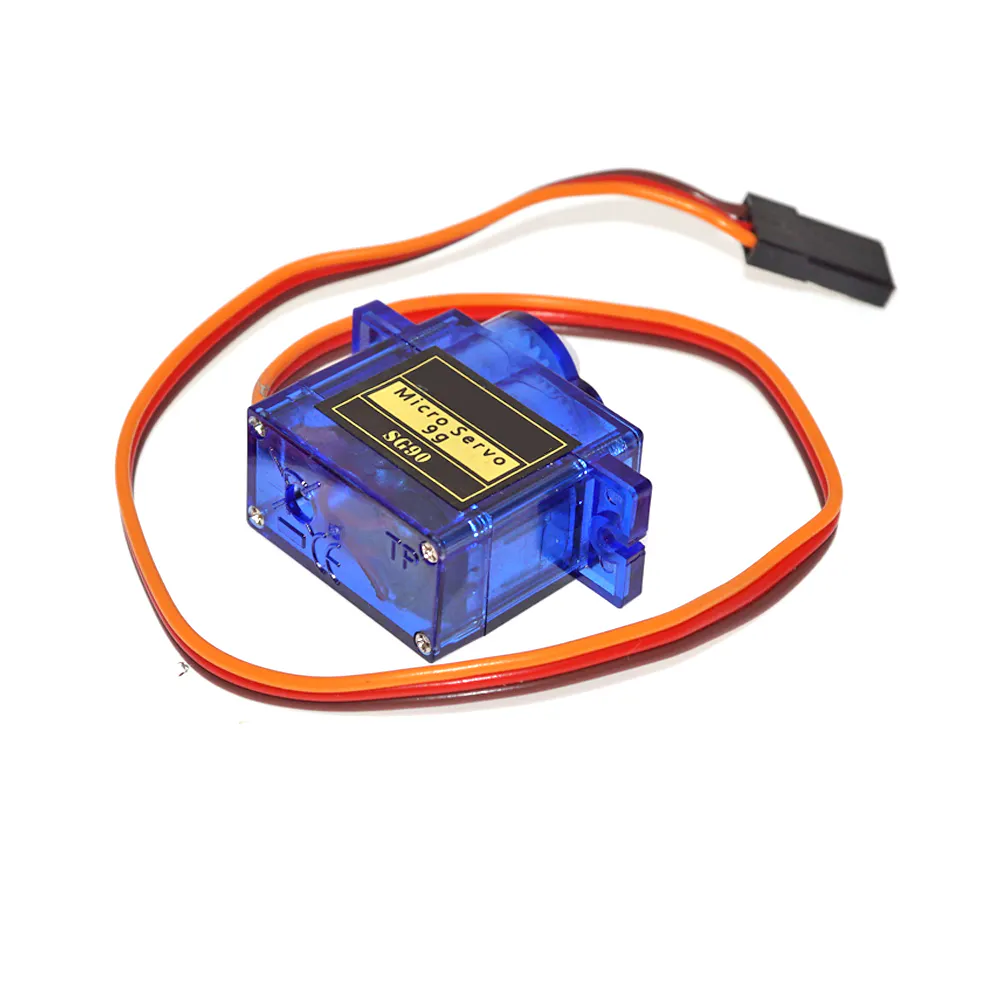SG90 Micro-servo motor
×1Ultrasonic Sensor - HC-SR04 (Generic)
×1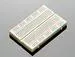×1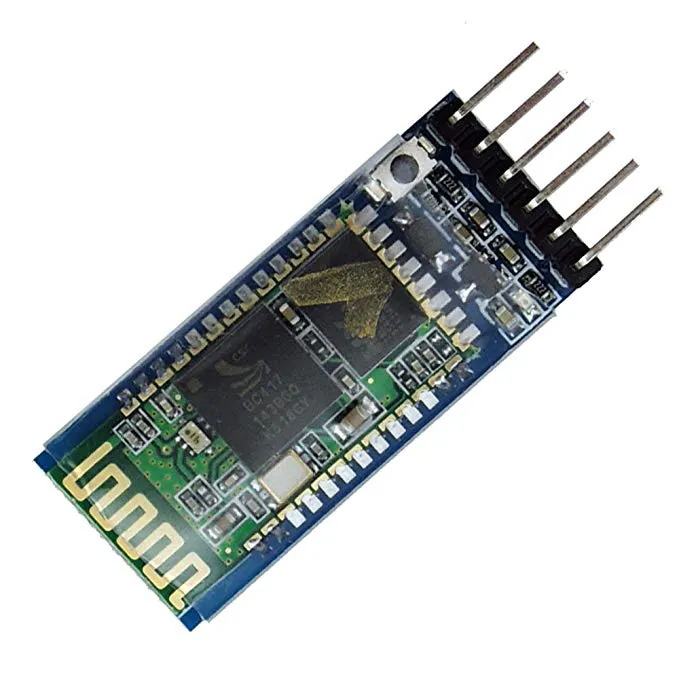HC-05 Bluetooth Module (Optional) Used to collect and display sensor readings
×1

## Schematics

### Basic Schematic

Note: Certain components are not shown as they were specific to this project. I find it more beneficial to all to see and understand the concepts behind the motor control and sornar system.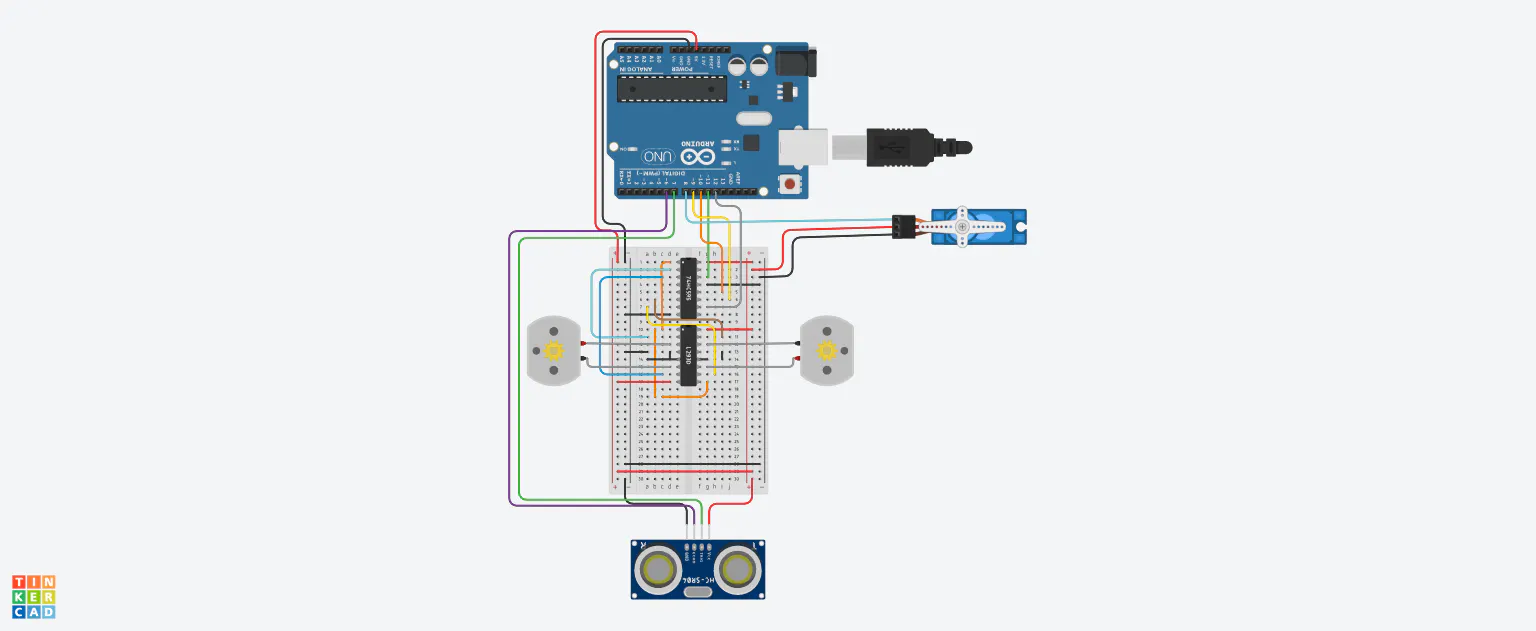## Code

### Full Code (ARDUINO IDE)

C/C++
NOTE: I didn't originally plan to share this code, so it's a little messy. You should still be able to get a general understanding of how the system works
```#include <Servo.h>

// ULTRASONIC SENSOR
const int trigPin = 7; //Define Trig pin
const int echoPin = 6; //Define Echo pin

//ARRAYS
static float dist_table;
static float pos_table;
int face_here = 0;

//SERVO
Servo servo;

//74HC595 PINS
int outputEnablePin = 12;//Pin connected to Output enable of 74HC595
int dataPin = 11; //Pin connected to DS of 74HC595
int latchPin = 10;//Pin connected to ST_CP of 74HC595
int clockPin = 9;//Pin connected to SH_CP of 74HC595

byte FWD = 0x46; //Foward
byte BCK = 0x8A; //Backwards
byte L = 0x4A; //Left
byte R = 0x86; //Right
byte CLEAR = 0x3; //Clear Register

byte data;
int delayTime;

int PWM=250;

void setup() {
pinMode(trigPin, OUTPUT);
pinMode(echoPin, INPUT);

pinMode(dataPin, OUTPUT);
pinMode(clockPin, OUTPUT);
pinMode(latchPin, OUTPUT);
pinMode(outputEnablePin, OUTPUT);

servo.attach(8);
Serial.begin(9600);
servo.write(87);

Clear_Reg();

//START SEQUENCE
delay(10000);
SonarscanA();
delay(50);
servo.write(87);
delay(50);
Positioning();
delay(50);
Short_Exploration();

}

void loop() {}

//Distance Measurments
float UltrasonicMeasuring () {
float duration, distance;
digitalWrite(trigPin, LOW); //Start low for 2 milliseconds
delayMicroseconds(2);
digitalWrite(trigPin, HIGH); //Sonic burst of 8 cycles for 10 milliseconds
delayMicroseconds(10);
digitalWrite(trigPin, LOW);
duration = pulseIn(echoPin, HIGH);
distance = (duration * .0343) / 2;
return distance;
}

//SCAN Process
float*SonarscanA() {
float distance;
float distA;
float distB;
int pos = 10;
int p = 20;
int i = 0;
int j = 0;
int prev = 0;
int check;
int RD;
servo.write(10);
delay(50);

for (pos = 10; pos <= 170; pos += 2) {
servo.write(pos);
Serial.print(pos);
Serial.print(",");
delayMicroseconds(50);
distA = UltrasonicMeasuring ();
delay(p);
distB = UltrasonicMeasuring ();
delay(p);
distance = (distA + distB) / 2;

RD = round(distance);
Serial.print(RD);
Serial.print(".");

dist_table[i] = distance;
pos_table[i] = pos;
i = i + 1;
}
for (pos = 170; pos >= 10; pos -= 2) {
servo.write(pos);
Serial.print(pos);
Serial.print(",");
delayMicroseconds(50);
distA = UltrasonicMeasuring ();
delay(p);
distB = UltrasonicMeasuring ();
delay(p);
distance = (distA + distB) / 2;

RD = round(distance);
Serial.print(RD);
Serial.print(".");

dist_table[81 - j] = (dist_table[81 - j] + distance) / 2;
j = j + 1;
}

for (int c = 0; c < 81; c++) {
check = dist_table[c];
if (check >= prev) {
face_here = pos_table[c];
prev = check;
}
}
return face_here;
}

//Determin direction to face after scan
void Positioning() {
if (face_here >= 0 && face_here <= 19) {
data = R;
delayTime = 510;
Register();
}
if (face_here >= 20 && face_here <= 39) {
data = R;
delayTime = 410;
Register();
}
if (face_here >= 40 && face_here <= 59) {
data = R;
delayTime = 310;
Register();
}
if (face_here >= 60 && face_here <= 79) {
data = R;
delayTime = 160;
Register();
}
if (face_here >= 80 && face_here <= 99) {
data = FWD;
delayTime = 100;
Register();
}
if (face_here >= 100 && face_here <= 119) {
data = L;
delayTime = 160;
Register();
}
if (face_here >= 120 && face_here <= 139) {
data = L;
delayTime = 310;
Register();
}
if (face_here >= 140 && face_here <= 159) {
data = L;
delayTime = 410;
Register();
}
if (face_here >= 160 && face_here <= 179) {
data = L;
delayTime = 510;
Register();
}
}

//Register
void shiftWrite(int desiredPin, boolean desiredState) {
bitWrite(data, desiredPin, desiredState);
shiftOut(dataPin, clockPin, MSBFIRST, data);
digitalWrite(latchPin, HIGH);
digitalWrite(latchPin, LOW);
}

void Register()
{
int index;
setpwm(PWM);
for (index = 0; index <= 7; index++) {
shiftWrite(index, HIGH);
delay(delayTime);
shiftWrite(index, LOW);
}
}

//TEST EXPLORATION
void Short_Exploration() {
int thresh = 15; //Sensor distance threshold
float distance;
int finish = 0;
int RD;
int pos = 90;
while (finish < 10) {  //As a test the robot completes 10 turns before stopping
Serial.print(pos);
Serial.print(",");
distance = UltrasonicMeasuring ();
RD = round(distance);
Serial.print(RD);
Serial.print(".");
if (distance < thresh) {
Clear_Reg();
Decide_Direction();
finish = finish + 1;
}
else {
Move_FWD();
}
}
}

//MOVE FOWARD Function
void Move_FWD() {
data = FWD;
digitalWrite(latchPin, 0);
shiftOut(dataPin, clockPin, data);
digitalWrite(latchPin, 1);
}

//Shifting bits into the register
void shiftOut(int myDataPin, int myClockPin, byte myDataOut) {
int i = 0;
int pinState;
pinMode(myClockPin, OUTPUT);
pinMode(myDataPin, OUTPUT);
digitalWrite(myDataPin, 0);
digitalWrite(myClockPin, 0);
for (i = 7; i >= 0; i--)  {
digitalWrite(myClockPin, 0);
if ( myDataOut & (1 << i) ) {
pinState = 1;
}
else {
pinState = 0;
}
digitalWrite(myDataPin, pinState);
digitalWrite(myClockPin, 1);
digitalWrite(myDataPin, 0);
}
digitalWrite(myClockPin, 0);
}

// Direction Decision
float Decide_Direction() {
float distA, distB;
float Left, Right;
float Turn;
int RD;
int l = 130;
int r = 50;
servo.write(130);
Serial.print(l);
Serial.print(",");
delay(350);
distA = UltrasonicMeasuring ();
delay(20);
distB = UltrasonicMeasuring ();
Left = (distA + distB) / 2;

RD = round(Left);
Serial.print(RD);
Serial.print(".");

servo.write(50);
Serial.print(r);
Serial.print(",");
delay(350);
distA = UltrasonicMeasuring ();
delay(20);
distB = UltrasonicMeasuring ();
Right = (distA + distB) / 2;

RD = round(Right);
Serial.print(RD);
Serial.print(".");

servo.write(87);

if (Left > Right) {
data = L;
delayTime = 350;
Register();
}
if (Right > Left) {
data = R;
delayTime = 350;
Register();
}
}

//CLAR REGISTER
void Clear_Reg() {
data = CLEAR;
delayTime = 150;
Register();
}

//PWM setup
void setpwm(byte pwm) // 0 to 255
{
analogWrite(outputEnablePin, 255-pwm);
}
```

### Processing IDE Code

Processing
This code was not originally made by me. Full credits to How To Mechatronics
```import processing.serial.*; // imports library for serial communication
import java.awt.event.KeyEvent; // imports library for reading the data from the serial port
import java.io.IOException;
Serial myPort; // defines Object Serial
// defubes variables
String angle="";
String distance="";
String data="";
String noObject;
float pixsDistance;
int iAngle, iDistance;
int index1=0;
int index2=0;
PFont orcFont;
void setup() {

size (1920, 1080); // ***CHANGE THIS TO YOUR SCREEN RESOLUTION***
smooth();
myPort = new Serial(this,"COM4", 9600); // starts the serial communication
myPort.bufferUntil('.'); // reads the data from the serial port up to the character '.'. So actually it reads this: angle,distance.

delay(1000);
}
void draw() {

fill(98,245,31);
textFont(orcFont);
// simulating motion blur and slow fade of the moving line
noStroke();
fill(0,4);
rect(0, 0, width, height-height*0.065);

fill(98,245,31); // green color
// calls the functions for drawing the radar
drawLine();
drawObject();
drawText();
}
void serialEvent (Serial myPort) { // starts reading data from the Serial Port
// reads the data from the Serial Port up to the character '.' and puts it into the String variable "data".
data = data.substring(0,data.length()-1);

index1 = data.indexOf(","); // find the character ',' and puts it into the variable "index1"
angle= data.substring(0, index1); // read the data from position "0" to position of the variable index1 or thats the value of the angle the Arduino Board sent into the Serial Port
distance= data.substring(index1+1, data.length()); // read the data from position "index1" to the end of the data pr thats the value of the distance

// converts the String variables into Integer
iAngle = int(angle);
iDistance = int(distance);
}
pushMatrix();
translate(width/2,height-height*0.074); // moves the starting coordinats to new location
noFill();
strokeWeight(2);
stroke(98,245,31);
// draws the arc lines
arc(0,0,(width-width*0.0625),(width-width*0.0625),PI,TWO_PI);
arc(0,0,(width-width*0.27),(width-width*0.27),PI,TWO_PI);
arc(0,0,(width-width*0.479),(width-width*0.479),PI,TWO_PI);
arc(0,0,(width-width*0.687),(width-width*0.687),PI,TWO_PI);
// draws the angle lines
line(-width/2,0,width/2,0);
popMatrix();
}
void drawObject() {
pushMatrix();
translate(width/2,height-height*0.074); // moves the starting coordinats to new location
strokeWeight(9);
stroke(255,10,10); // red color
pixsDistance = iDistance*((height-height*0.1666)*0.025); // covers the distance from the sensor from cm to pixels
// limiting the range to 40 cms
if(iDistance<15){
// draws the object according to the angle and the distance
}
popMatrix();
}
void drawLine() {
pushMatrix();
strokeWeight(9);
stroke(30,250,60);
translate(width/2,height-height*0.074); // moves the starting coordinats to new location
popMatrix();
}
void drawText() { // draws the texts on the screen

pushMatrix();
if(iDistance>10) {
noObject = "Out of Range";
}
else {
noObject = "In Range";
}
fill(0,0,0);
noStroke();
rect(0, height-height*0.0648, width, height);
fill(98,245,31);
textSize(25);

text("10cm",width-width*0.3854,height-height*0.0833);
text("20cm",width-width*0.281,height-height*0.0833);
text("30cm",width-width*0.177,height-height*0.0833);
text("40cm",width-width*0.0729,height-height*0.0833);
textSize(40);
text("Object: " + noObject, width-width*0.875, height-height*0.0277);
text("Angle: " + iAngle +" °", width-width*0.48, height-height*0.0277);
text("Distance: ", width-width*0.26, height-height*0.0277);
if(iDistance<10) {
text("        " + iDistance +" cm", width-width*0.225, height-height*0.0277);
}
textSize(25);
fill(98,245,60);
text("30°",0,0);
resetMatrix();
text("60°",0,0);
resetMatrix();
text("90°",0,0);
resetMatrix();
text("120°",0,0);
resetMatrix();